# Pass list as command line argument in Python

The arguments that are given after the name of the program in the command line shell of the operating system are known as Command Line Arguments. Python provides various ways of dealing with these types of arguments. One of them is `sys` module.

## sys Module

A module is a file containing Python definitions and statements. The sys module provides functions and variables used to manipulate different parts of the Python runtime environment. This module provides access to some variables used or maintained by the interpreter and to functions that interact strongly with the interpreter.

#### sys.argv

`sys.argv` is used in python to retrieve command line arguments at runtime. For a program to be able to use it, it has to be imported from the “sys” module. The arguments so obtained are in the form of an array named `sys.argv`.

Note: `sys.argv` gives the name of the program and the following indices work like members of an array.

Approach:
The name of the program is “cmdlist.py”.

1. Working with command line: Consider the below code written in cmdlis.py

 `import` `sys ` ` `  ` `  `print``(``"the name of the program is "``, sys.argv[``0``]) ` `print``(``"argument list :"``, sys.argv) `

Output: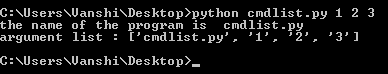2. Calling a list using the command line

 `import` `sys ` ` `  ` `  `print` `(``"the name of the program is "``, sys.argv[``0``]) ` ` `  `a ``=` `sys.argv[``1``] ` `print` `(a) `

Output: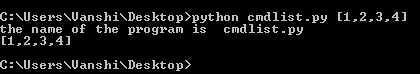3. Working with the list called by command line.
sys.argv is a string array. Thus, any argument passed to it is a string so in order to properly put in use we have to convert it to an appropriate list form.

• Program to take list as command line argument and convert it to a proper list form

 `import` `sys ` ` `  ` `  `print``(``"the name of the program is "``, sys.argv[``0``]) ` ` `  `n ``=` `len``(sys.argv[``1``]) ` `a ``=` `sys.argv[``1``][``1``:n``-``1``] ` `a ``=` `a.split(``', '``) ` ` `  `for` `i ``in` `a: ` `    ``print``(i) `

Output: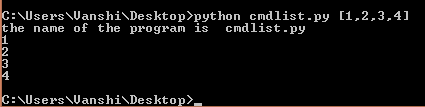• Program to find the sum of all the members of the list
Also, keep in find to change the members to the required data type if not string.

 `import` `sys ` ` `  ` `  `print` `(``"the name of the program is"``, sys.argv[``0``]) ` ` `  `n``=``len``(sys.argv[``1``]) ` `a``=``sys.argv[``1``][``1``:n``-``1``] ` `a``=``a.split(``','``) ` ` `  `A ``=` `[``int``(i) ``for` `i ``in` `a] ` ` `  `b ``=` `0` ` `  `for` `i ``in` `A: ` `    ``b ``+``=` `i ` `print``(``"sum of all the list members is "``, b) `

Output: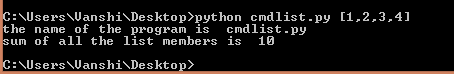4. Working with multiple list retrieved through command line.

 `import` `sys ` ` `  ` `  `print` `(``"the name of the program is "``, sys.argv[``0``]) ` ` `  `n ``=` `len``(sys.argv[``1``]) ` `a ``=` `sys.argv[``1``][``1``:n``-``1``] ` `a ``=` `a.split(``', '``) ` ` `  `A ``=` `[``int``(i) ``for` `i ``in` `a] ` `b ``=` `0` ` `  `for` `i ``in` `A: ` `    ``b ``+``=` `i ` `print``(``"sum of all the list members is "``, b) ` ` `  `n ``=` `len``(sys.argv[``2``]) ` `c ``=` `sys.argv[``2``][``1``:n``-``1``] ` `c ``=` `c.split(``', '``) ` ` `  `d ``=` `"" ` ` `  `for` `i ``in` `c: ` `    ``d ``=` `d``+``i ` ` `  `print` `(``"sum of all the list members is "``, d) `

Output: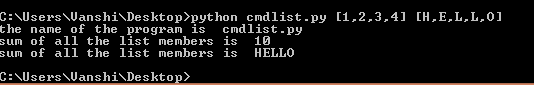My Personal Notes arrow_drop_upCheck out this Author's contributed articles.

If you like GeeksforGeeks and would like to contribute, you can also write an article using contribute.geeksforgeeks.org or mail your article to contribute@geeksforgeeks.org. See your article appearing on the GeeksforGeeks main page and help other Geeks.

Please Improve this article if you find anything incorrect by clicking on the "Improve Article" button below.

Article Tags :

Be the First to upvote.

Please write to us at contribute@geeksforgeeks.org to report any issue with the above content.